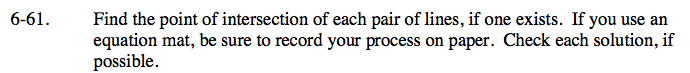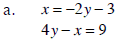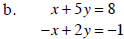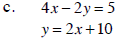### Home > CAAC > Chapter 6 > Lesson 6.2.3 > Problem6-61

6-61.
1. Find the point of intersection of each pair of lines, if one exists. If you use an equation mat, be sure to record your process on paper. Check each solution, if possible. 6-61a HW eTool (CPM), 6-61b HW eTool (CPM) and 6-61c HW eTool (CPM). Homework Help ✎

1. x = −2y −3
4yx = 9

2. x + 5y = 8
x + 2y = −1

3. 4x − 2y = 5
y = 2x + 10Use the substitution method:
4y − (−2y − 3) = 9

Combine like terms and solve. Then substitute the y value you found into the first equation and solve for x.Use the elimination method. Solve for y and substitute the value into one of the equations.

x = 3 and y = 1See problem (a) for help.

Use the eTools below to help solve the problems.

Click the link at right for the full version of the eTool: AC 6-61a HW eTool

Click the link at right for the full version of the eTool: AC 6-61b HW eTool

Click the link at right for the full version of the eTool: AC 6-61c HW eTool